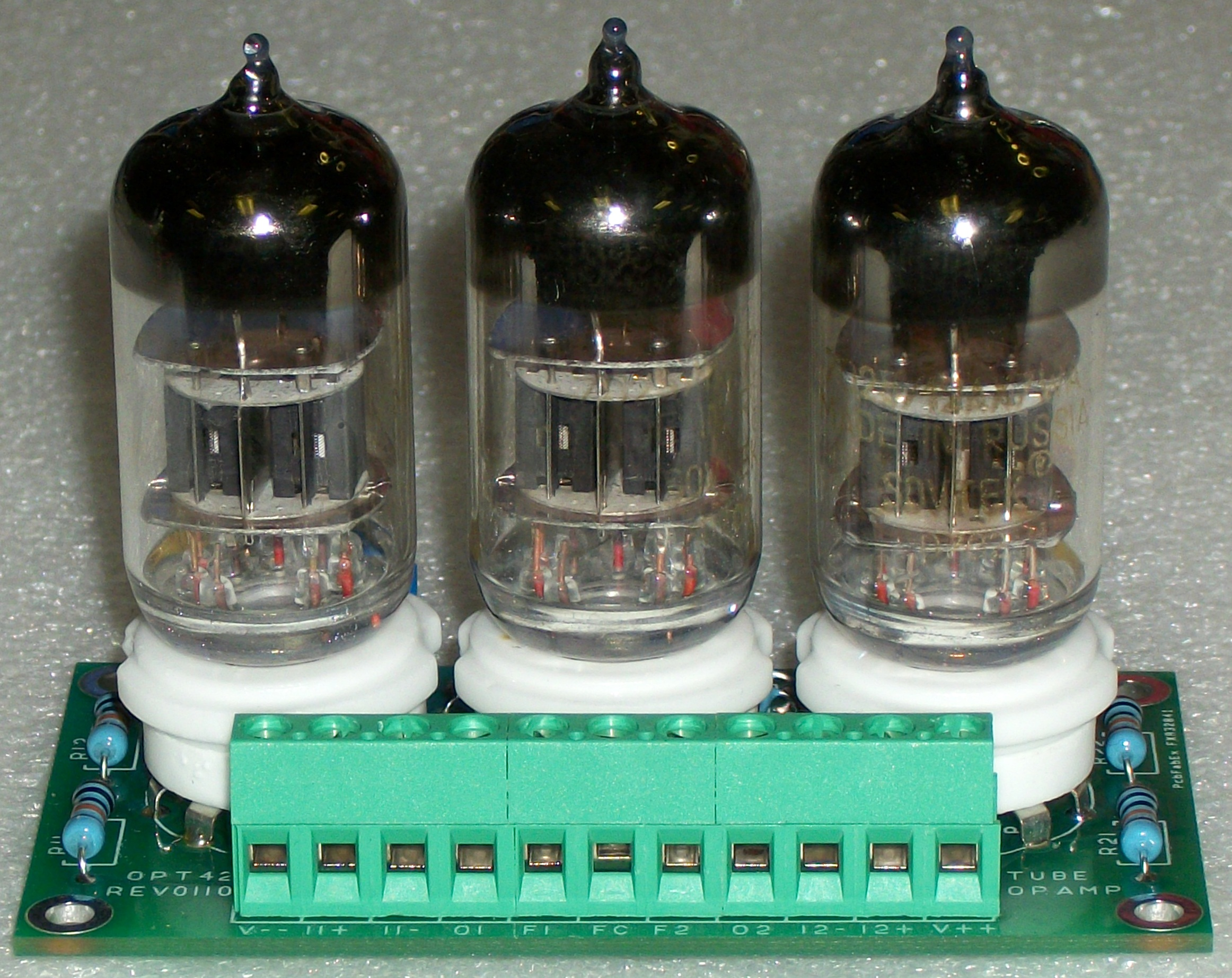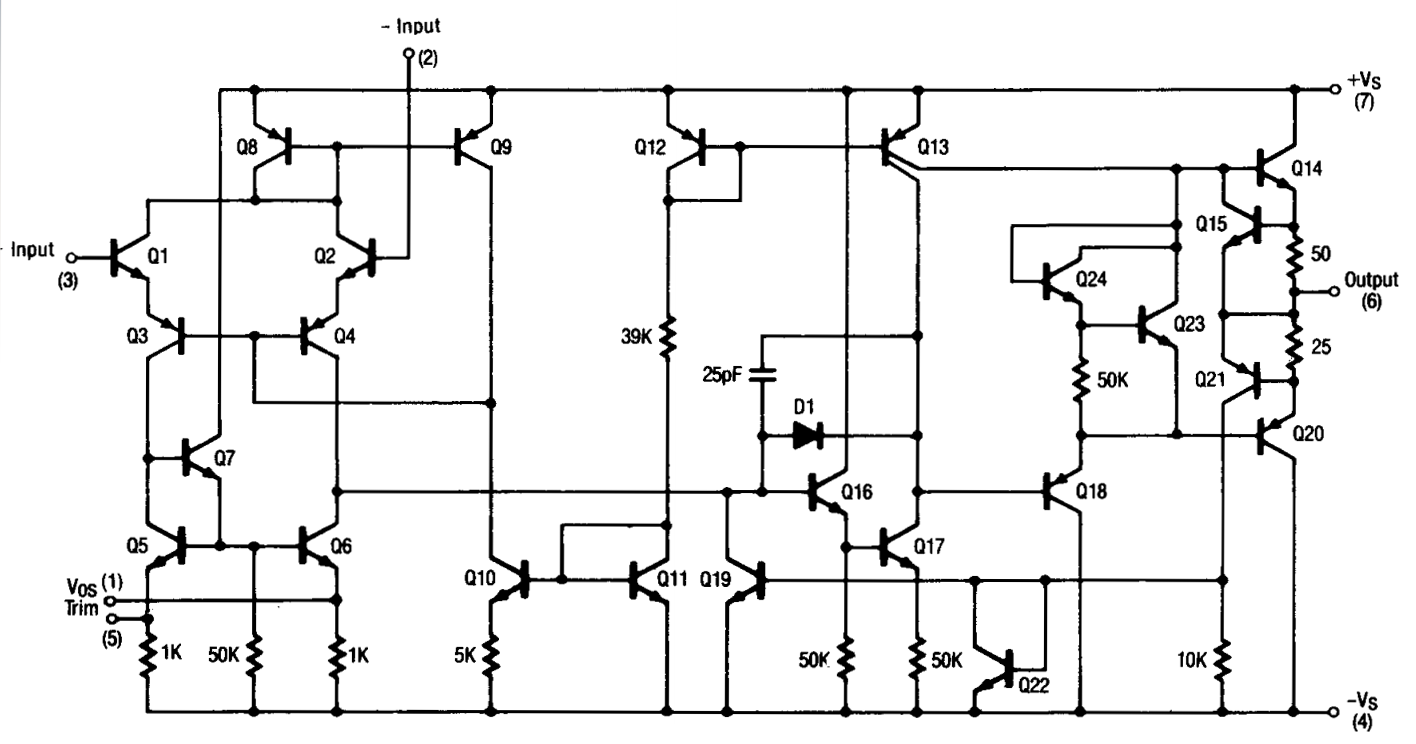Tutorial Analog Computing - [Part 1: LM741] filter_list Author Message
Analog Computing - [Part 1: LM741] #1
My probe didn't yield very many results, but I'm really bored, so I figured I might as well cover some basic concepts.

In this installation, we'll be talking about the integrated circuit LM741, AKA the Operational Amplifier (OpAmp). Back in the day this was a vacuum tube, and looked something like the followingOf course now they're made as discrete semiconductors, out of digital components, and internally look more like thisNow, the next thing that might jump out to you is the fact that I've put labels on two resistors in this circuit. Those are actually critical to computing, the entire purpose of the computer depends on these resistors. They're what actually do the work (sort of), not the amps themselves. Without these resistors, we end up with this:No matter what voltage we give it, we'll always get either + or -15 volts. These resistors are what prevents this from happening. The effect is simply infinite gain (up to the limits of the amplifier)

We're going to briefly go over a single application of these, to do basic mathematical operations, as well as design a couple circuits. Everything will be simulated on falstad simulator, and I will provide demo links.

Okay, let's jump into it. What are operational amplifiers? Well, to be short (I'm not going into the electrical engineering behind these in this series), they're amplifier circuits that can utilize a feedback loop to do operations. In this thread, we'll simply be working with the following basic diagram (link):So, looking at the above image, we can see that this circuit has turned our +15 volt supply into -15 volts. This is a key observation to make. The LM741, when connected like this (most common application here) is in inversion mode, meaning that it will invert the sum of its operated inputs (that phrase will be important later on). If we put -15v into it, we'd get +15v out of it.

It's also key to remember that in analog computing, we aren't dealing with 1s and 0s, we're dealing with voltage, usually in the range of -15 to +15 volts DC, though this can easily be whatever you feel like, so long as your OpAmps can handle it. For our purposes, we'll stick simply with the standard 30-volt range.

The next thing you may have noticed are the two labeled resistors. These are critical and are what make the OpAmp do useful work. Without these resistors, we would simply get infinite gain, and would always be at the maximum voltage of the device. In our case, 15 volts either positive or negative.So, let's talk about the first operation we can do with these. Multiplication. I know it's backwards, you're all used to digital stuff where you do addition first and multiplication is much more difficult (when it's by a factor other than 2). Let's go ahead and multiply our input voltage by 3. To do this, I'm just going to set the power supply voltage to 4, since we don't have any inputs yet, and then I'm going to change the values of the resistors. This is the result (link):Other than changing the supply voltage from +15v to +4v, I only changed R1 to be 3k Ohms. You can see that at the output we're getting -12v DC, or the equivalent of multiplying by -3. This was the important bit I talked about earlier, anything we put in will get inverted. We'll come back to that in just a moment.
So, why did changing R1 give me multiplication? Well, that's actually a pretty simple answer. For a single input device like this, the output is clamped to
Code:
`Input * (R1 / R2) * -1`
So, by that logic, we have
Code:
`4 * (3 / 1) * -1 = -12`

So, what if we don't want to multiply by -1? For the scope of this thread, we can't get around that, but that doesn't mean that we have to accept a negative answer, we just need to craft a second stage that multiplies by -1. To do that, we need R1/R2 to be 1, and simply feed it the output of our first stage.
This just means that R1 and R2 need to be the same value. They can be any value, usually they're increments of 10 because those resistors are easy to find, so we'll go ahead and make everything in increments of 10 and wire it up. What we end up with looks like this.I've added boxes around the individual components so that it's easier to see what's going on, and I've labeled the inputs and outputs of each part. From here on, we'll be using the following naming structure for pins:
Code:
```A, B, C, D = inputs Q = output```
Since this is a single input function, you're looking for A for the input to each box, and Q for the output. You can see that in the first box, A=4 and Q=-12, then it goes straight into the second such that A=-12 and Q=12.
Congrats! We've just made a hardware implementation of the function Q=3*A using only 6 components, at a cost of a few pennies.

So, what about addition? What if we wanted to make the function Q=A+B? Well, that's where it starts to get a little weirder and we need some extra components. I won't go into the theory behind why this is acceptable, but just understand that this is the ONLY case that it's acceptable to do something like this. Take a look at the following (link):So, the gist of why we can connect them together like that is that at the point we connect the wires, there is only a minute voltage, so it's ground for all intensive purposes, and you can always connect ground to ground. You can verify this in the simulator by hovering over that junction. I get 90uV.

And that's the end of this tutorial, feel free to play around with things in the simulator, and don't hesitate to post your designs and circuits. The first person to implement the following function in its least cost form (lowest number of components) using what I've shown you here will get the remainder of my NSP.
Code:
`Q=(1/2A)+(2B)`
Excluding the power supplies, the smallest circuit should use 7 components. Have fun.

Users browsing this thread: 1 Guest(s)